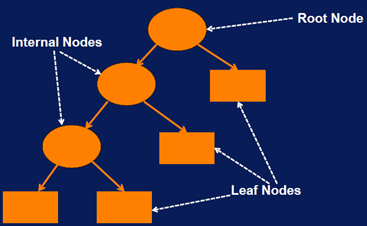## 1. Definition:

• Decision tree overview:
• Idea: Split data into “pure” regions (regions with samples from only one class)
• Each subset contains as many samples as possible from a single class.
• Boundaries separating these regions are called decision boundaries. And the decision tree model makes classification based on these decision boundaries.
• Classification using decision tree:• The root and internal nodes have test conditions.
• Each leaf node has a class label associated with it.
• A classification decision is made by traversing the decision tree starting with the root node. At each node the answer to the test condition determines which branch to traverse to. When a leaf node is reached the category at the leaf node determines the classification decision.
• Depth of a node: The number of edges from the root node to that node.
• The depth of the root node is 0.
• The depth of a decision tree is the number of edges in the longest path from the root node to the leaf node.
• The size of a decision tree is the number of nodes in the tree.
• Constructing decision tree:
• Start with all samples at a node.
• Partition samples based on input to create purest subsets
• Repeat to partition data into successively purer subsets
• Induction algorithm: Algorithm for constructing a decision tree model (or tree induction).
• Greedy approach:
• The tree has to be built in piecemeal fashion by determining the best way to split the current node at each step. And combining these decisions together to form the final decision tree.
• It turns out that it works out better mathematically if we measure the impurity rather than the purity of a split.
• Impurity measure = specifies how mixed the resulting subsets are.
• A common impurity measure used for determining the best split is the Gini index.
• The lower the Gini Index the higher the purity of the split.
• Other impurity measures include entropy, or information gain, and misclassification rate.
• What variable to split on:
• Splits on all variables are tested using a purity measure such as the Gini index to compare the various possibilities.
• When to stop splitting a node:
• All (or X% of) samples have same class label.
• Number of samples in node reaches minimum.
• Change in impurity measure is smaller than threshold.
• Max tree depth is reached.
• Others….